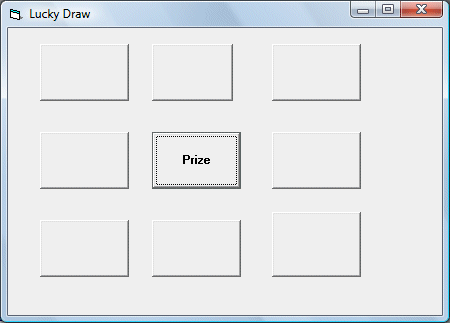# Lucky Draw Program

This is a program that simulates a lucky draw game. This program consists of  a 3x3 matrix, which means there are 9 command buttons in a grid. The command buttons are created as controls in an array, and they are differentiated by their indices. One of the button contains a prize,  when you click on it, it  displays the word "prize" on the caption. If you do not  strike the prize , the word "The Prize is here! " will appear on the command button that contains the prize.

We use a randomize process to create the chance events. Random integers from 1 to 9 can be created using the statement n=Int(Rnd*9)+1. Int is a function that converts numbers to integers and Rnd is a method that generates random numbers between 0 and 1. When the user clicks a command button, a random number between 0 and 9 is generated. If this number corresponds to the index of the command button, it will show the word "Prize" on the clicked command button  , otherwise, it shows the words "The Prize is here!" on the command button with an index corresponds to the generated number.

### The Interface### The Code

Private Sub Command1_Click(Index As Integer)
Dim n As Integer
For n = 0 To 8
Command1(n).Caption = ""
Next
n = Int(Rnd * 9)+1
If Index = n Then
Command1(n).Caption = "Prize"
Else: Command1(n).Caption = "The Prize is here!"
End If

End Sub